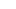# CAT Score VS CAT Percentile – Understand the big differenceThe Common Aptitude Test (CAT) is one of the most important management entrance tests and is conducted by. The CAT is a national level exam which is taken by lakhs of aspirants.

Because of its popularity, it was quite difficult to conduct examinations all over India at the same time; therefore, the concept of taking examinations at different times with different question papers was introduced.

The introduction of this new topic leads to a new system of marking and evaluating. Let go through this simple article to calculate marks and clear the basic doubts faced by aspiring students.

Understanding the marking scheme of the CAT exam is extremely crucial for the candidates. The exam comprises of a total of 100 questions which have been divided into three sectors.

Every correct answer is rewarded +3 marks while every wrong answer attracts a penalty of -1 marks. Any un-attempted questions are not awarded any marks. The final CAT score obtained is out of a total of 300comprising of the three sections of 100 marks each.

The CAT score and CAT marks are the same. But percentile has a completely different meaning and is calculated by formulas. It reflects your performance in the exam when compared with other fellow candidates who also took the same exam.

For e.g., you have scored a 75 percentile in your CAT, that means you have scored better than 75 per cent of the total appearing students and only 15% scored more than you. Hence you should not confuse between CAT score, which is the measure of your score with respect to the question paper vs.

CAT percentile which is a measure of your score with respect to a whole lot of other students. Thus, it can be safely analysed that instead of reflecting how the candidate has performed in the exam, CAT percentile reflects the relative performance of the candidate in comparison with the other candidates.

## How to calculate CAT Percentile

Here are all the steps that are involved in the process of calculation of CAT percentile: –

1. Assume the total number of students appearing for the exam to be “N.” This will include students from both the sessions, i.e. morning and afternoon.
2. Based on the scaled score, a rank is assigned to each candidate. Thereafter a normalisation process is conducted wherein a specific “r” rank based on the scaled score is awarded.
3. Now, the following formula is used to calculate the CAT Percentile based on the above-mentioned data,

P= [( N – r )/ N] x 100,

Here, P represents the percentile of the candidate, N represents the total number of candidates and r reflects the rank of the candidate.

As different entrance exams are conducted by business schools across India, it becomes necessary to ensure equality. That is why a scaled score is applied, which considers the performance of the candidates across the sessions as well as the difficulty of the exam.

This helps in normalisation of the score, and it ensures that candidates across both sessions are able to compete in a similar manner. Hope this helps you clear your doubts on CAT scores and percentiles.

Stay connected with fellow students on PaGaLGuY for CAT Exam 2020 Discussion